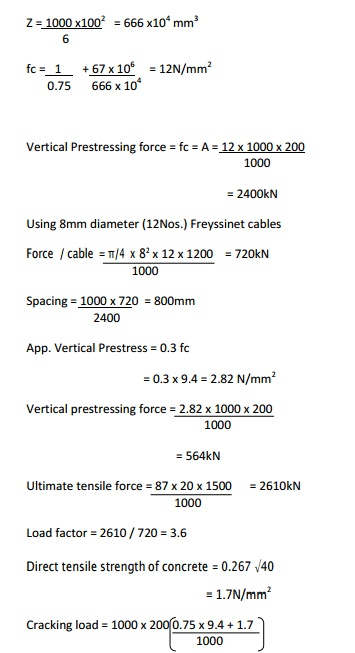Home | | Prestressed Concrete Structures | Design Procedure for circular tanks Computations

# Design Procedure for circular tanks Computations

1. Minimum wall thickness 2. Circumferential Prestress 3. Vertical Prestress.

Design Procedure for circular tanks Computations

1. Minimum wall thickness

2. Circumferential Prestress

3. Vertical Prestress.

Estimate

1)    Maximum, ring tension Nd

2)     Bending Moment Mw

3)     Minimum wall thickness = Nd/?fct - fmin.w

- Minimum cover 35mm

4)    Circumferential Prestress5)    Spacing of wires

As = Cross sectional area of wire coinding, mm2

Wt = average radial Pressure of wires at transfer at a given section N/mm2

D = Diameter of the tank, mm

S = Spacing of wires at the given section mm

fs - Stress in wires at transfer, N/mm2

t - Thickness of the tank wall, mm

fc - compressive stress in concrete, N/mm2

Hoop compression due toprestressing = wt . D / 2

Equating

wt. D/2 = fs As/S1

Wt = 2 fs As / sD

Nd - hoop tension due to hydrostatic working pressure, Ww

Nt - hoop compression due to radial pressure of wires, wt

Then Nt = Nd (wt/Ww)

Also Nt = t fc

Spacing of the wire winding

S = (2 Nd/ Ww)  . ( fs./fc )  .( As/Dt)  mm

Mt = Mw (wt /Ww)

Where Mt = Vertical moment due to the prestress at transfer.

Mw = Vertical moment due to hydrostatic pressure.

The compressive prestress required

Fc =(  fmin. W / ? ) +  ( Mw/ ?z)

When the tank is empty

Fc =(  fmin. W / ? ) +  ( Mw/Z)

Vertical prestressing force is required

P = fc. Ac

(Note: Vertical Prestressing force = 30% of hoop compression.]

1.     A cylindrical prestressed concrete water tank of internal diameter 30m is required to store water over a depth of 7.5m. The permissible compressive stress in concrete at transfer is 13 and the minimum compressive stress under working presuure is 1 N/mm2. . The loss ratio is 0.75. Wires of 5mm diameter with an initial stress of 1000N/mm2  are available for circumferential winding and freyssinet cables made up of 12 wires of 8mm diameter stressed to 1200N/mm2 are to be used for vertical prestressing. Design the tank walls assuming the base as fixed. The cube strength of concrete is 40N/mm2.Study Material, Lecturing Notes, Assignment, Reference, Wiki description explanation, brief detail
Civil : Prestressed Concrete Structures : Circular Prestressing : Design Procedure for circular tanks Computations |